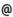## "Babes-Bolyai" University of Cluj-Napoca Faculty of Mathematics and Computer Science

 Geometry 3
 Code Semes-ter Hours: C+S+L Credits Type Section MMG0004 3 2+2+0 6 compulsory Matematică MMG0004 3 2+2+0 5 compulsory Matematică informatică MMG0004 3 2+2+0 6 compulsory Matematici aplicate
 Teaching Staff in Charge
 Prof. VARGA Csaba Gyorgy, Ph.D., csvargacs.ubbcluj.ro
 Assoc.Prof. BLAGA Paul Aurel, Ph.D., pablagacs.ubbcluj.ro
 Aims The course gives to the students the principal tools and also the necesary methods in the study of curves and surfaces with an accent on the intuitive side.
 Content I. Local theory of curves. 1. Curves in euclidean space.Tangent line and normal plane. 2. Curves in general position. Osculating plane. 3. Frenet frame. Frenet formulas. Curvature and torsion. 4. Geometric iterpretation of curvature and torsion. 5. Contact beatween two curves. 6. Evolute and involute. 7. Envelope of a family of curves in plane. II.Local theory of surfaces. 1. Surfaces in three-dimensional euclidean space. 2. Tangent plane and normal line of a surface. 3. First fundamental form of a surface. 4. Arc length , angle between two curves on surface .Area element. 5. Second fundamental form. 6. Normal curvature. 7. Asymptotic lines of a surface. 8. Principal curvatutres of a surface. Mean curvature and Gaussian curvature. 9. Theorema egregium. 10.Minimal surfaces and surfaces with constant curvature. 11.Darboux frame. Darboux formulas. 12.Geodesic curvature. Geodesic torsion. 13.Geodesic lines.
 References 1. BAER, C.: Elementare differentialgeometrie, Walter de Gruyter, 2001 2. DO CARMO, M.P.: Differential Geometry of Curves and Surfaces, Prentice Hall, 1976 3. FEDENKO, A.S.: Culegere de probleme de geometrie diferentiala (în limba rusa), Nauka, Moscova, 1979 4. GRAY, A.: Modern Differential Geometry of Curves and Surfaces, CRC Press, 1993 5. HSIUNG, C.C.: A First Course in Differential Geometry, John Wiley, 1981 6. KLINGENBERG, W: A Course in Differential Geometry, Springer, 1983 7. KUEHNEL, W.: Differentialgeometrie, Vieweg, 1999 8. MILLMAN, R. - PARKER, G.: Elements of Differential Geometry, Prentice Hall, 1977 9. OPREA, J.: Differential Geometry and its Applications, Prentice Hall, 1997 10. STOKER, J.J.: Differential Geometry, John Wiley, 1969 11. STRUIK, D.J.: Lectures on Classical Differential Geometry, Dover, 1988 12. TAJMANOV, I.A.: Lectii de geometrie diferentiala (în limba rusa), Editura IKI, Moscova, 2002
 Assessment 30% from the final mark is the activity during one semester 70% from the final mark is the mark from a written test.